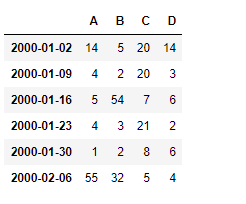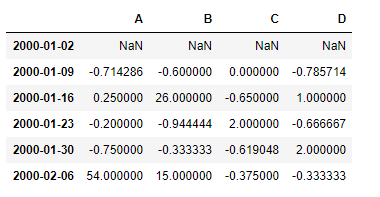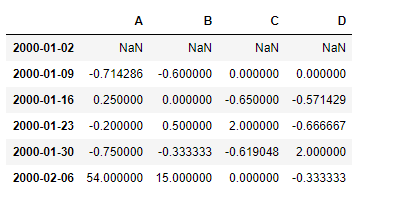# Python | Pandas dataframe.pct_change()

Python is a great language for doing data analysis, primarily because of the fantastic ecosystem of data-centric python packages. Pandas is one of those packages and makes importing and analyzing data much easier.

Pandas` dataframe.pct_change()` function calculates the percentage change between the current and a prior element. This function by default calculates the percentage change from the immediately previous row.

Note : This function is mostly useful in the time-series data.

Syntax: DataFrame.pct_change(periods=1, fill_method=’pad’, limit=None, freq=None, **kwargs)

Parameters :
periods : Periods to shift for forming percent change.
fill_method : How to handle NAs before computing percent changes.
limit : The number of consecutive NAs to fill before stopping
freq : Increment to use from time series API (e.g. ‘M’ or BDay()).
**kwargs : Additional keyword arguments are passed into DataFrame.shift or Series.shift.

Returns : The same type as the calling object.

Example #1: Use `pct_change()` function to find the percentage change in the time-series data.

 `# importing pandas as pd ` `import` `pandas as pd ` ` `  `# Creating the time-series index ` `ind ``=` `pd.date_range(``'01/01/2000'``, periods ``=` `6``, freq ``=``'W'``) ` ` `  `# Creating the dataframe  ` `df ``=` `pd.DataFrame({``"A"``:[``14``, ``4``, ``5``, ``4``, ``1``, ``55``], ` `                   ``"B"``:[``5``, ``2``, ``54``, ``3``, ``2``, ``32``],  ` `                   ``"C"``:[``20``, ``20``, ``7``, ``21``, ``8``, ``5``], ` `                   ``"D"``:[``14``, ``3``, ``6``, ``2``, ``6``, ``4``]}, index ``=` `ind) ` ` `  `# Print the dataframe ` `df `Let’s use the `dataframe.pct_change()` function to find the percent change in the data.

 `# find the percentage change with the previous row ` `df.pct_change() `

Output :The first row contains `NaN` values, as there is no previous row from which we can calculate the change.

Example #2: Use `pct_change()` function to find the percentage change in the data which is also having `NaN` values.

 `# importing pandas as pd ` `import` `pandas as pd ` ` `  `# Creating the time-series index ` `ind ``=` `pd.date_range(``'01/01/2000'``, periods ``=` `6``, freq ``=``'W'``) ` ` `  `# Creating the dataframe  ` `df ``=` `pd.DataFrame({``"A"``:[``14``, ``4``, ``5``, ``4``, ``1``, ``55``], ` `                   ``"B"``:[``5``, ``2``, ``None``, ``3``, ``2``, ``32``],  ` `                   ``"C"``:[``20``, ``20``, ``7``, ``21``, ``8``, ``None``], ` `                   ``"D"``:[``14``, ``None``, ``6``, ``2``, ``6``, ``4``]}, index ``=` `ind) ` ` `  `# apply the pct_change() method ` `# we use the forward fill method to ` `# fill the missing values in the dataframe ` `df.pct_change(fill_method ``=``'ffill'``) `

Output :The first row contains `NaN` values, as there is no previous row from which we can calculate the change. All the `NaN` values in the dataframe has been filled using `ffill` method.

My Personal Notes arrow_drop_upCheck out this Author's contributed articles.

If you like GeeksforGeeks and would like to contribute, you can also write an article using contribute.geeksforgeeks.org or mail your article to contribute@geeksforgeeks.org. See your article appearing on the GeeksforGeeks main page and help other Geeks.

Please Improve this article if you find anything incorrect by clicking on the "Improve Article" button below.

Article Tags :

Be the First to upvote.

Please write to us at contribute@geeksforgeeks.org to report any issue with the above content.